# Frank Solutions for Class 10 Maths Chapter 8 Reflection

Frank Solutions for Class 10 Maths Chapter 8 Reflection are available here. Our expert faculty formulated these solutions to assist students in the board exam preparation and attain good marks in Maths. While solving the exercise questions from the Frank textbook, students often face difficulty and eventually pile up their doubts. Hence, students are advised that they practise Frank Solutions for Class 10 Maths to clear their doubts so that they can score good marks in the subject.

Chapter 8 – Reflection: Reflection is known as a flip. A reflection is a mirror image of the shape. An image will reflect through a line, known as the reflection. When a figure is said to be a reflection of the other figure, every point in the figure is equidistant from each corresponding point in another figure. Students will learn to find the coordinates of the images and more concepts in Chapter 8, Reflection.

## Download the PDF of Frank Solutions for Class 10 Maths Chapter 8 Reflection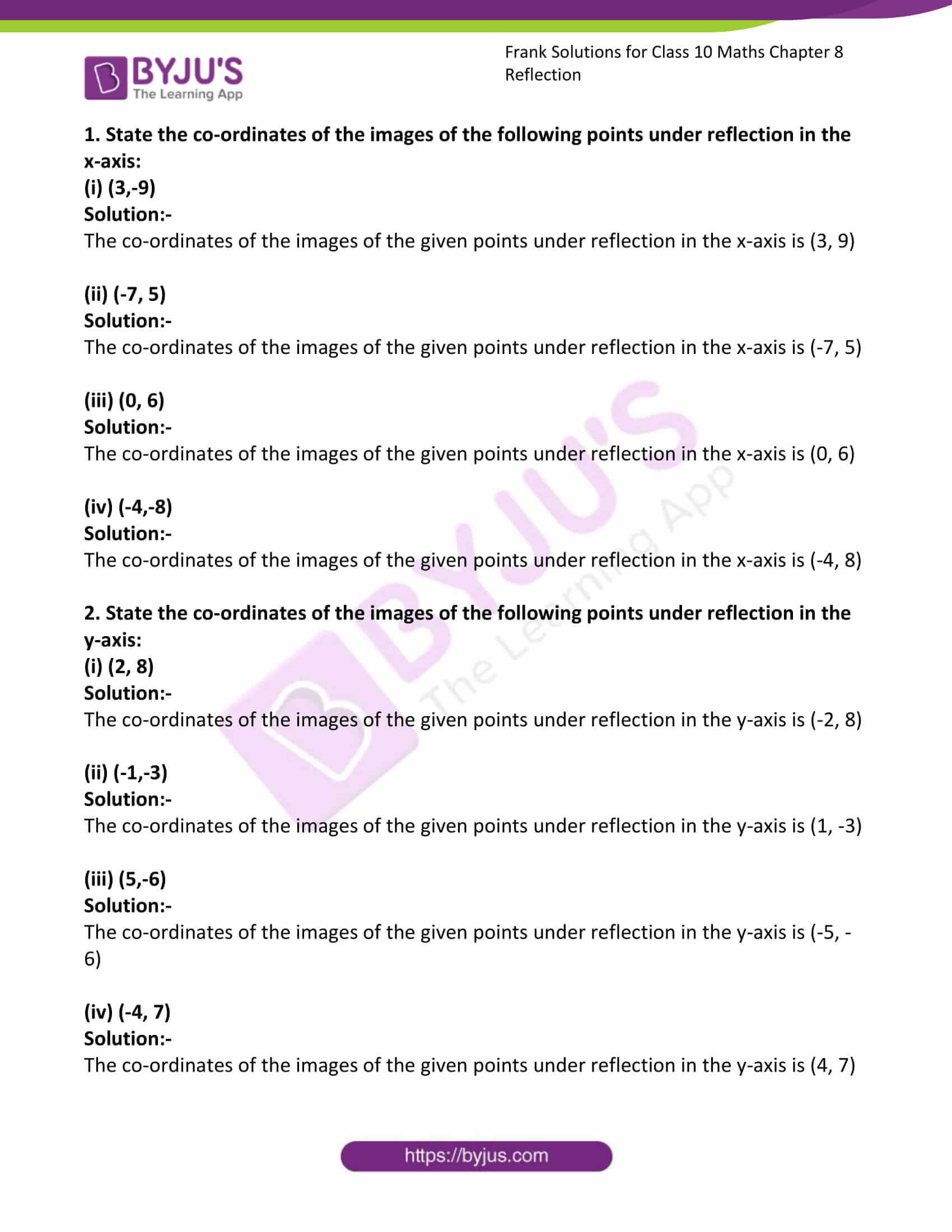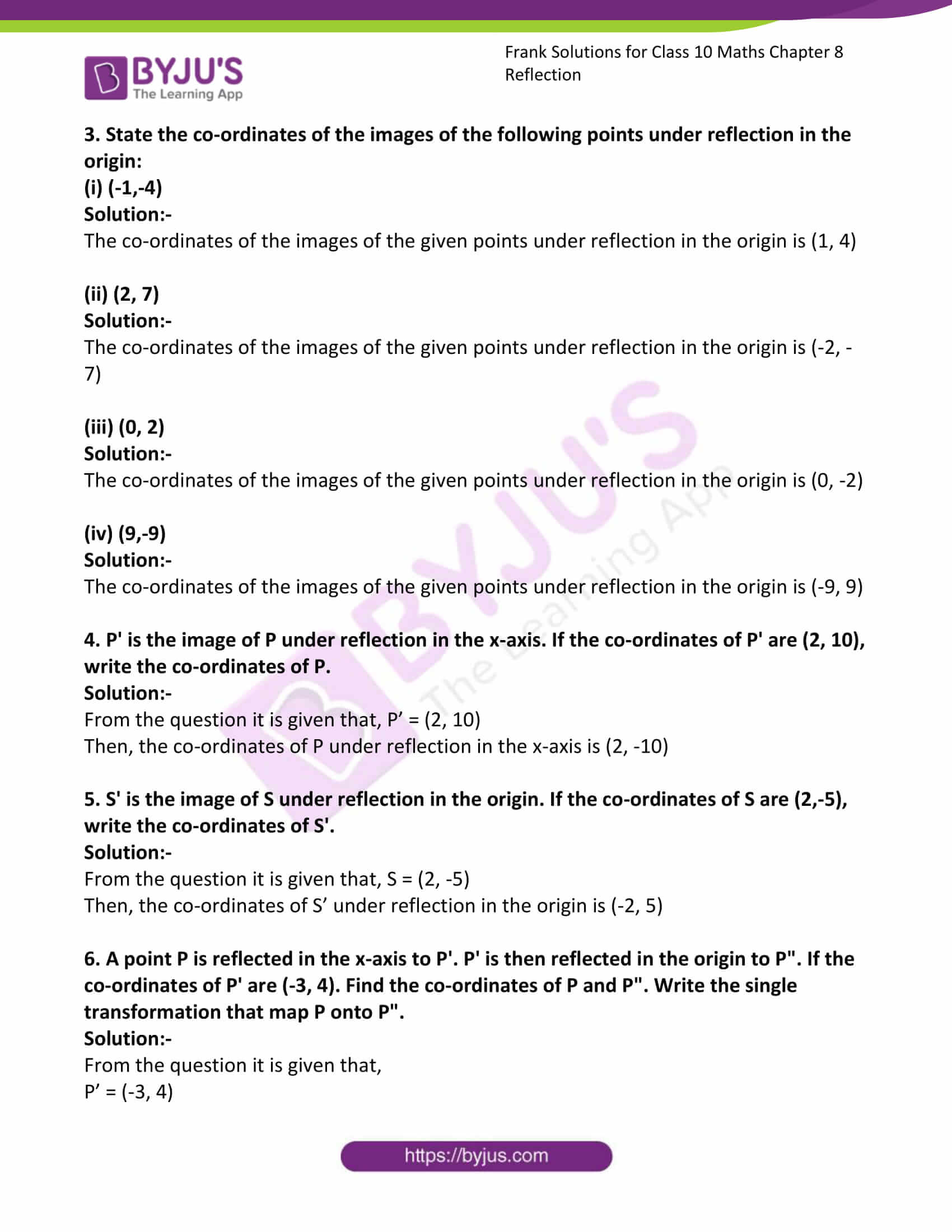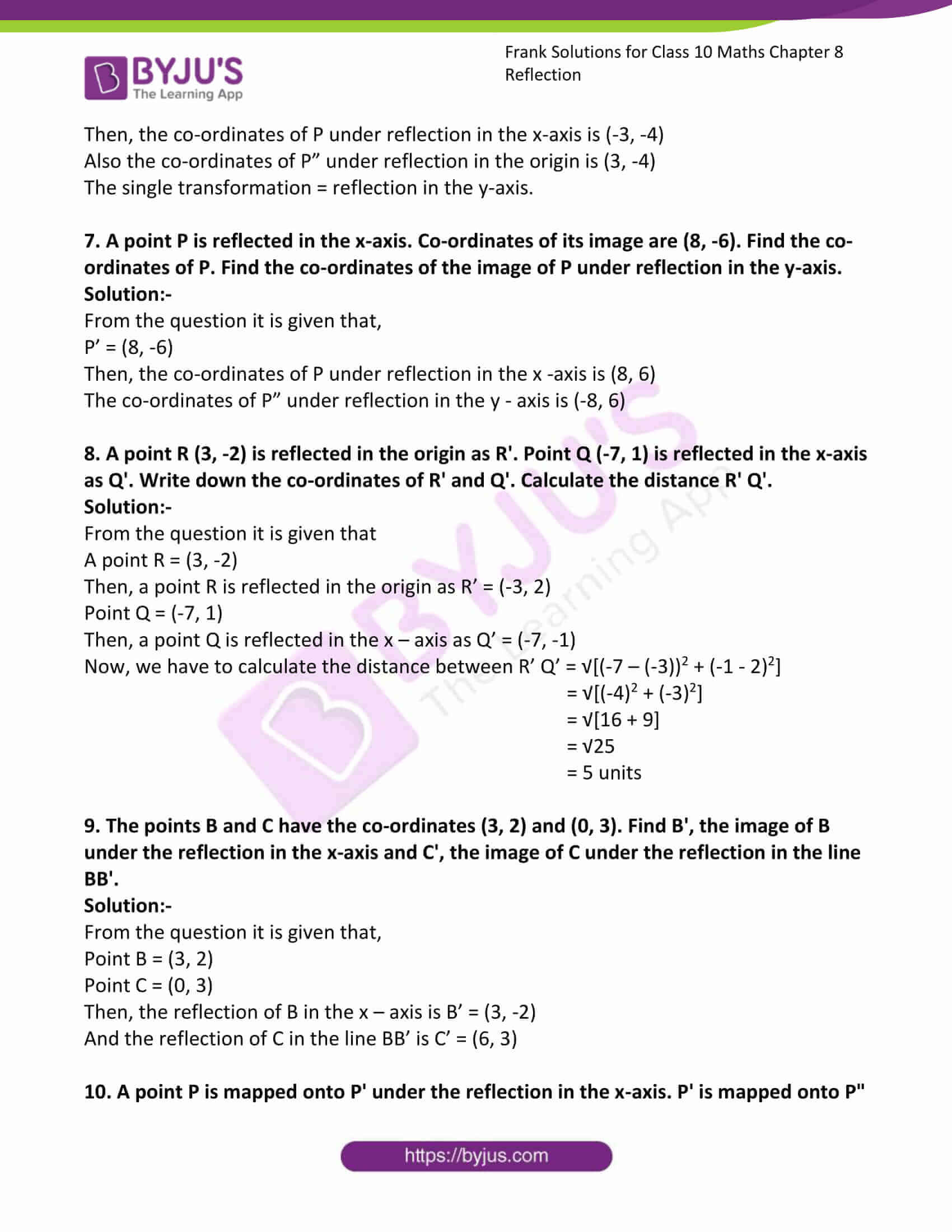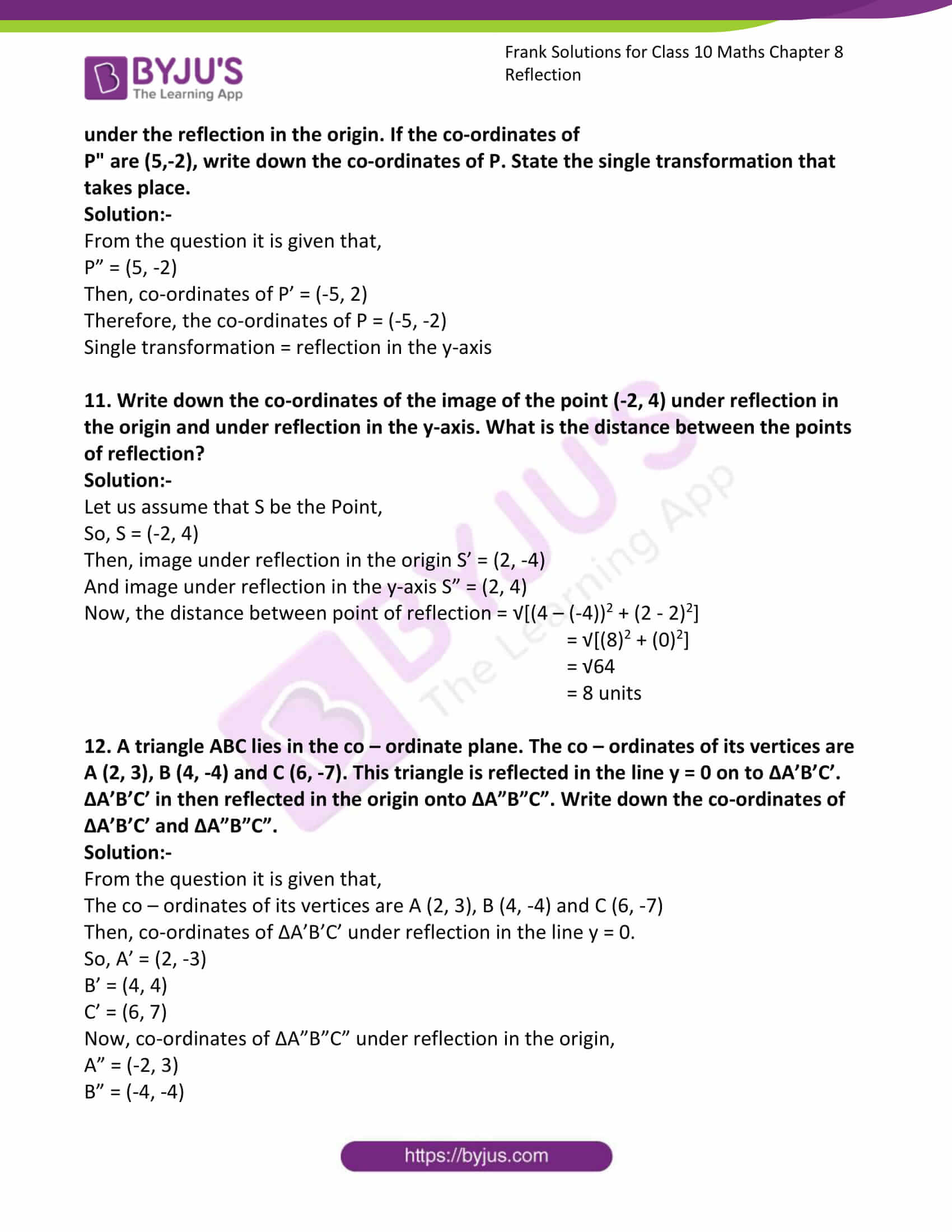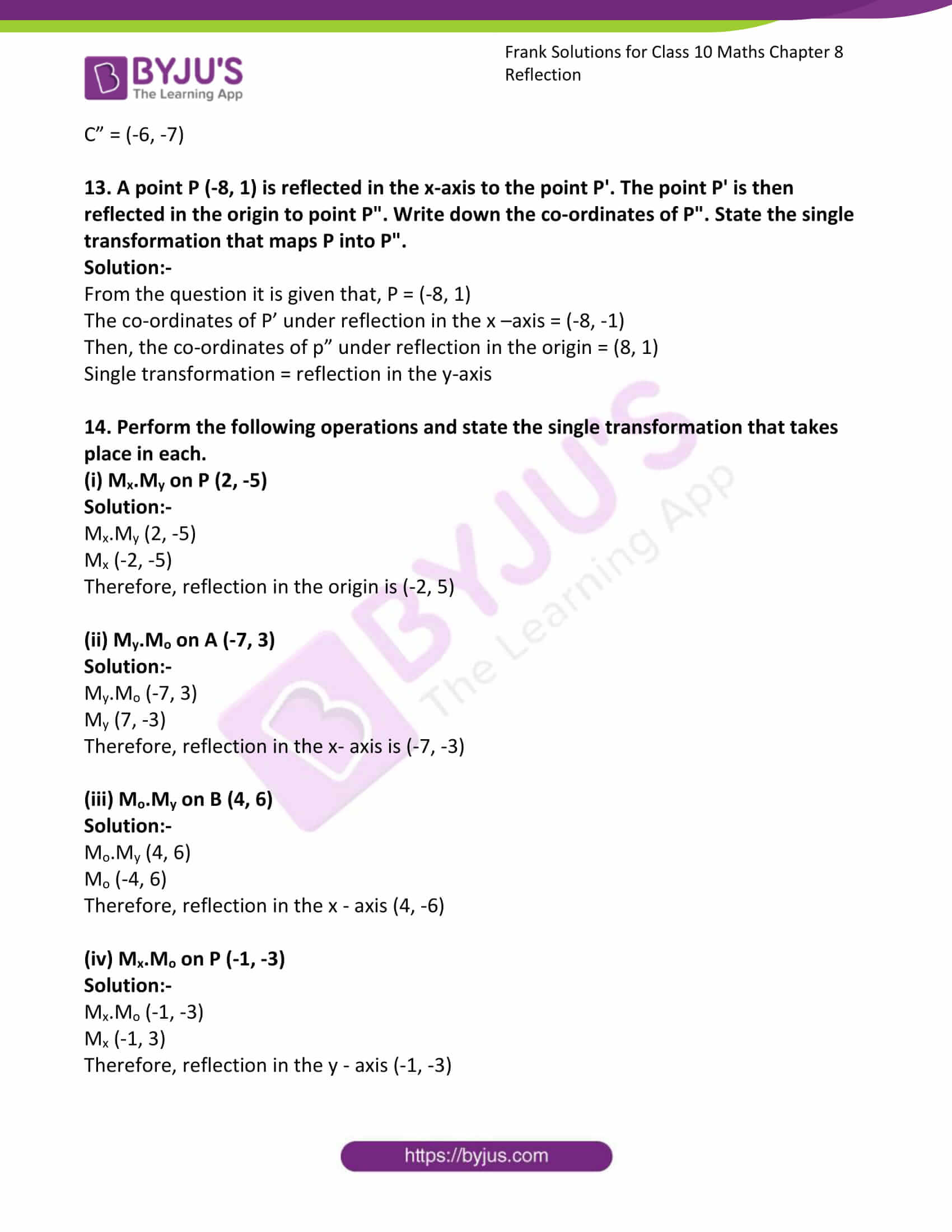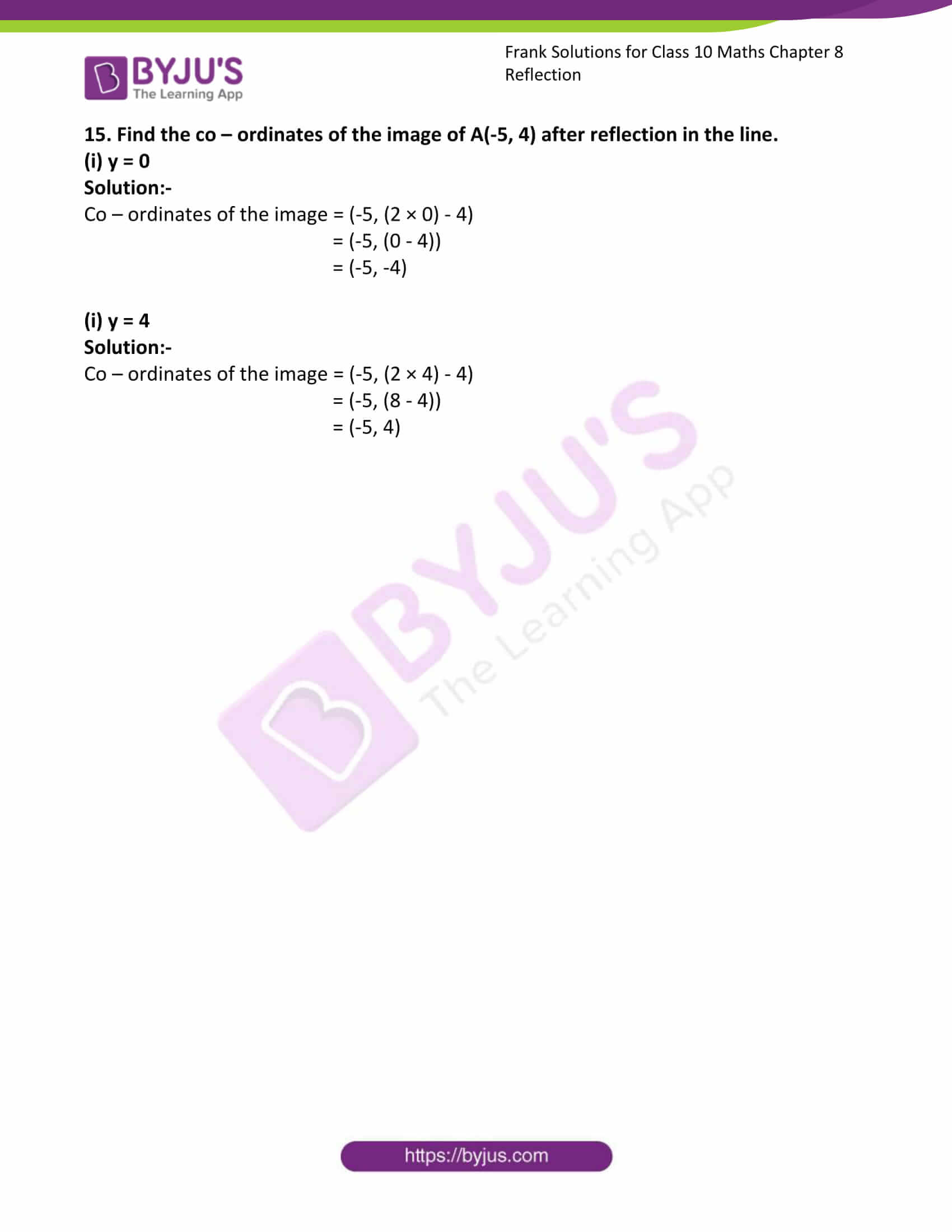### Access Answers to Frank Solutions for Class 10 Maths Chapter 8 Reflection

1. State the co-ordinates of the images of the following points under reflection in the x-axis:
(i) (3,-9)

Solution:-

The co-ordinates of the images of the given points under reflection in the x-axis are (3, 9)

(ii) (-7, 5)

Solution:-

The co-ordinates of the images of the given points under reflection in the x-axis are (-7, 5)

(iii) (0, 6)

Solution:-

The co-ordinates of the images of the given points under reflection in the x-axis are (0, 6)

(iv) (-4,-8)

Solution:-

The co-ordinates of the images of the given points under reflection in the x-axis are (-4, 8)

2. State the co-ordinates of the images of the following points under reflection in the y-axis:
(i) (2, 8)

Solution:-

The co-ordinates of the images of the given points under reflection in the y-axis are (-2, 8)

(ii) (-1,-3)

Solution:-

The co-ordinates of the images of the given points under reflection in the y-axis are (1, -3)

(iii) (5,-6)

Solution:-

The co-ordinates of the images of the given points under reflection in the y-axis are (-5, -6)

(iv) (-4, 7)

Solution:-

The co-ordinates of the images of the given points under reflection in the y-axis are (4, 7)

3. State the co-ordinates of the images of the following points under reflection in origin:
(i) (-1,-4)

Solution:-

The co-ordinates of the images of the given points under reflection in origin are (1, 4)

(ii) (2, 7)

Solution:-

The co-ordinates of the images of the given points under reflection in origin are (-2, -7)

(iii) (0, 2)

Solution:-

The co-ordinates of the images of the given points under reflection in origin are (0, -2)

(iv) (9,-9)

Solution:-

The co-ordinates of the images of the given points under reflection in origin are (-9, 9)

4. P’ is the image of P under reflection in the x-axis. If the co-ordinates of P’ are (2, 10), write the co-ordinates of P.

Solution:-

From the question, it is given that, P’ = (2, 10)

Then, the co-ordinates of P under reflection in the x-axis is (2, -10)

5. S’ is the image of S under reflection in origin. If the co-ordinates of S are (2,-5), write the co-ordinates of S’.

Solution:-

From the question, it is given that, S = (2, -5)

Then, the co-ordinates of S’ under reflection in origin is (-2, 5)

6. A point P is reflected in the x-axis to P’. P’ is then reflected in origin to P”. If the co-ordinates of P’ are (-3, 4). Find the co-ordinates of P and P”. Write the single transformation that maps P onto P”.

Solution:-

From the question, it is given that,

P’ = (-3, 4)

Then, the co-ordinates of P under reflection in the x-axis is (-3, -4)

Also, the co-ordinates of P” under reflection in origin is (3, -4)

The single transformation = reflection in the y-axis.

7. A point P is reflected in the x-axis. Co-ordinates of its image are (8, -6). Find the co-ordinates of P. Find the co-ordinates of the image of P under reflection in the y-axis.

Solution:-

From the question, it is given that,

P’ = (8, -6)

Then, the co-ordinates of P under reflection in the x-axis is (8, 6)

The co-ordinates of P” under reflection in the y-axis is (-8, 6)

8. A point R (3, -2) is reflected in origin as R’. Point Q (-7, 1) is reflected in the x-axis as Q’. Write down the co-ordinates of R’ and Q’. Calculate the distance R’ Q’.

Solution:-

From the question, it is given that

A point R = (3, -2)

Then, a point R is reflected in origin as R’ = (-3, 2)

Point Q = (-7, 1)

Then, a point Q is reflected in the x-axis as Q’ = (-7, -1)

Now, we have to calculate the distance between R’ Q’ = √[(-7 – (-3))2 + (-1 – 2)2]

= √[(-4)2 + (-3)2]

= √[16 + 9]

= √25

= 5 units

9. Points B and C have the co-ordinates (3, 2) and (0, 3). Find B’, the image of B under the reflection in the x-axis and C’, the image of C under the reflection in the line BB’.

Solution:-

From the question, it is given that,

Point B = (3, 2)

Point C = (0, 3)

Then, the reflection of B in the x-axis is B’ = (3, -2)

And the reflection of C in the line BB’ is C’ = (6, 3)

10. A point P is mapped onto P’ under the reflection in the x-axis. P’ is mapped onto P” under the reflection in origin. If the co-ordinates of P” are (5,-2), write down the co-ordinates of P. State the single transformation that takes place.

Solution:-

From the question, it is given that,

P” = (5, -2)

Then, co-ordinates of P’ = (-5, 2)

Therefore, the co-ordinates of P = (-5, -2)

Single transformation = reflection in the y-axis

11. Write down the co-ordinates of the image of the point (-2, 4) under reflection in origin and under reflection in the y-axis. What is the distance between the points of reflection?

Solution:-

Let us assume that S be the Point,

So, S = (-2, 4)

Then, image under reflection in origin S’ = (2, -4)

And image under reflection in the y-axis S” = (2, 4)

Now, the distance between point of reflection = √[(4 – (-4))2 + (2 – 2)2]

= √[(8)2 + (0)2]

= √64

= 8 units

12. A triangle ABC lies in the co-ordinate plane. The co-ordinates of its vertices are A (2, 3), B (4, -4) and C (6, -7). This triangle is reflected in the line y = 0 on to ΔA’B’C’. ΔA’B’C’ is then reflected in origin onto ΔA”B”C”. Write down the co-ordinates of ΔA’B’C’ and ΔA”B”C”.

Solution:-

From the question it is given that,

The co-ordinates of its vertices are A (2, 3), B (4, -4) and C (6, -7)

Then, co-ordinates of ΔA’B’C’ under reflection in the line y = 0.

So, A’ = (2, -3)

B’ = (4, 4)

C’ = (6, 7)

Now, co-ordinates of ΔA”B”C” under reflection in origin,

A” = (-2, 3)

B” = (-4, -4)

C” = (-6, -7)

13. A point P (-8, 1) is reflected in the x-axis to the point P’. The point P’ is then reflected in origin to point P”. Write down the co-ordinates of P”. State the single transformation that maps P into P”.

Solution:-

From the question, it is given that, P = (-8, 1)

The co-ordinates of P’ under reflection in the x-axis = (-8, -1)

Then, the co-ordinates of p” under reflection in origin = (8, 1)

Single transformation = reflection in the y-axis

14. Perform the following operations and state the single transformation that takes place in each.

(i) Mx.My on P (2, -5)

Solution:-

Mx.My (2, -5)

Mx (-2, -5)

Therefore, reflection in origin is (-2, 5)

(ii) My.Mo on A (-7, 3)

Solution:-

My.Mo (-7, 3)

My (7, -3)

Therefore, reflection in the x-axis is (-7, -3)

(iii) Mo.My on B (4, 6)

Solution:-

Mo.My (4, 6)

Mo (-4, 6)

Therefore, reflection in the x-axis (4, -6)

(iv) Mx.Mo on P (-1, -3)

Solution:-

Mx.Mo (-1, -3)

Mx (-1, 3)

Therefore, reflection in the y-axis (-1, -3)

15. Find the co-ordinates of the image of A(-5, 4) after reflection in the line.

(i) y = 0

Solution:-

Co-ordinates of the image = (-5, (2 × 0) – 4)

= (-5, (0 – 4))

= (-5, -4)

(i) y = 4

Solution:-

Co-ordinates of the image = (-5, (2 × 4) – 4)

= (-5, (8 – 4))

= (-5, 4)